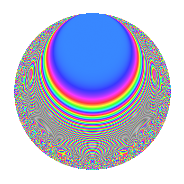# Properties

 Label 287.2.f.aLevel 287 Weight 2 Character orbit 287.f Analytic conductor 2.292 Analytic rank 0 Dimension 40 CM No

# Related objects

## Newspace parameters

 Level: $$N$$ = $$287 = 7 \cdot 41$$ Weight: $$k$$ = $$2$$ Character orbit: $$[\chi]$$ = 287.f (of order $$4$$ and degree $$2$$)

## Newform invariants

 Self dual: No Analytic conductor: $$2.29170653801$$ Analytic rank: $$0$$ Dimension: $$40$$ Relative dimension: $$20$$ over $$\Q(i)$$ Sato-Tate group: $\mathrm{SU}(2)[C_{4}]$

## $q$-expansion

The dimension is sufficiently large that we do not compute an algebraic $$q$$-expansion, but we have computed the trace expansion.

 $$\operatorname{Tr}(f)(q) =$$ $$40q + 4q^{3} - 36q^{4} + 8q^{6} + O(q^{10})$$ $$\operatorname{Tr}(f)(q) =$$ $$40q + 4q^{3} - 36q^{4} + 8q^{6} - 32q^{10} - 8q^{11} + 16q^{12} + 16q^{13} - 8q^{15} + 28q^{16} + 20q^{17} - 12q^{18} - 20q^{19} + 4q^{22} + 16q^{23} - 12q^{24} - 40q^{25} - 20q^{26} - 20q^{27} - 12q^{29} + 4q^{30} + 32q^{34} + 4q^{35} - 16q^{38} + 64q^{40} + 16q^{41} + 32q^{42} + 8q^{44} + 72q^{45} - 24q^{47} - 40q^{48} - 64q^{51} - 96q^{52} + 8q^{53} + 52q^{54} - 8q^{55} - 88q^{57} - 36q^{58} + 48q^{59} + 52q^{60} - 8q^{63} - 84q^{64} - 44q^{65} + 56q^{66} + 40q^{67} - 60q^{68} + 28q^{69} - 8q^{70} + 20q^{71} + 80q^{72} - 20q^{75} - 4q^{76} + 12q^{78} - 12q^{79} + 16q^{81} - 52q^{82} + 40q^{83} + 8q^{85} + 80q^{86} + 96q^{88} - 8q^{89} - 20q^{92} - 64q^{93} + 52q^{94} + 68q^{96} - 60q^{97} - 4q^{98} - 36q^{99} + O(q^{100})$$

## Embeddings

For each embedding $$\iota_m$$ of the coefficient field, the values $$\iota_m(a_n)$$ are shown below.

For more information on an embedded modular form you can click on its label.

Label $$a_{2}$$ $$a_{3}$$ $$a_{4}$$ $$a_{5}$$ $$a_{6}$$ $$a_{7}$$ $$a_{8}$$ $$a_{9}$$ $$a_{10}$$
50.1 2.46506i 0.589206 + 0.589206i −4.07653 2.39319i 1.45243 1.45243i 0.707107 + 0.707107i 5.11877i 2.30567i −5.89935
50.2 2.24636i −1.79418 1.79418i −3.04612 1.17005i −4.03037 + 4.03037i −0.707107 0.707107i 2.34997i 3.43817i −2.62835
50.3 1.99257i 1.04836 + 1.04836i −1.97032 1.71001i 2.08892 2.08892i −0.707107 0.707107i 0.0591377i 0.801887i −3.40730
50.4 1.72512i 2.12657 + 2.12657i −0.976043 0.471226i 3.66859 3.66859i 0.707107 + 0.707107i 1.76645i 6.04458i −0.812921
50.5 1.55423i −0.820177 0.820177i −0.415620 1.07119i −1.27474 + 1.27474i −0.707107 0.707107i 2.46249i 1.65462i 1.66487
50.6 1.43253i −1.43055 1.43055i −0.0521511 4.15342i −2.04931 + 2.04931i 0.707107 + 0.707107i 2.79036i 1.09294i −5.94992
50.7 1.13705i 1.99666 + 1.99666i 0.707122 2.87203i 2.27029 2.27029i −0.707107 0.707107i 3.07813i 4.97327i 3.26564
50.8 1.08678i −0.429589 0.429589i 0.818911 2.95294i −0.466868 + 0.466868i 0.707107 + 0.707107i 3.06353i 2.63091i 3.20919
50.9 0.447748i 1.09022 + 1.09022i 1.79952 1.12660i 0.488146 0.488146i 0.707107 + 0.707107i 1.70123i 0.622822i −0.504431
50.10 0.124298i −0.249942 0.249942i 1.98455 1.52864i −0.0310673 + 0.0310673i −0.707107 0.707107i 0.495271i 2.87506i −0.190006
50.11 0.701559i 2.17674 + 2.17674i 1.50781 3.99632i −1.52711 + 1.52711i −0.707107 0.707107i 2.46094i 6.47641i 2.80365
50.12 0.710667i 0.708215 + 0.708215i 1.49495 3.05444i −0.503305 + 0.503305i 0.707107 + 0.707107i 2.48375i 1.99686i −2.17069
50.13 0.748721i −1.37188 1.37188i 1.43942 2.22584i 1.02716 1.02716i 0.707107 + 0.707107i 2.57516i 0.764137i 1.66653
50.14 0.865152i −1.36546 1.36546i 1.25151 1.87589i 1.18133 1.18133i −0.707107 0.707107i 2.81305i 0.728936i −1.62293
50.15 1.38058i 1.24569 + 1.24569i 0.0939893 1.25269i −1.71978 + 1.71978i −0.707107 0.707107i 2.89093i 0.103480i −1.72944
50.16 1.70500i −1.24229 1.24229i −0.907032 2.38000i 2.11811 2.11811i 0.707107 + 0.707107i 1.86351i 0.0865659i −4.05791
50.17 2.30053i 1.78480 + 1.78480i −3.29242 0.414800i −4.10598 + 4.10598i 0.707107 + 0.707107i 2.97325i 3.37102i 0.954258
50.18 2.36496i −0.345245 0.345245i −3.59302 3.38746i 0.816490 0.816490i −0.707107 0.707107i 3.76741i 2.76161i 8.01120
50.19 2.69233i −2.23891 2.23891i −5.24863 1.01652i 6.02789 6.02789i 0.707107 + 0.707107i 8.74637i 7.02546i 2.73681
50.20 2.74225i 0.521769 + 0.521769i −5.51991 4.13490i −1.43082 + 1.43082i −0.707107 0.707107i 9.65245i 2.45551i −11.3389
See all 40 embeddings
 $$n$$: e.g. 2-40 or 990-1000 Embeddings: e.g. 1-3 or 155.20 Significant digits: Format: Complex embeddings Normalized embeddings Satake parameters Satake angles

## Inner twists

This newform does not have CM; other inner twists have not been computed.

## Hecke kernels

There are no other newforms in $$S_{2}^{\mathrm{new}}(287, [\chi])$$.